Transformers - 2

# Transformers - 2 Notes | Study Electrical Engineering SSC JE (Technical) - Electrical Engineering (EE)

## Document Description: Transformers - 2 for Electrical Engineering (EE) 2022 is part of Electrical Engineering SSC JE (Technical) preparation. The notes and questions for Transformers - 2 have been prepared according to the Electrical Engineering (EE) exam syllabus. Information about Transformers - 2 covers topics like Practical Transformer and Transformers - 2 Example, for Electrical Engineering (EE) 2022 Exam. Find important definitions, questions, notes, meanings, examples, exercises and tests below for Transformers - 2.

Introduction of Transformers - 2 in English is available as part of our Electrical Engineering SSC JE (Technical) for Electrical Engineering (EE) & Transformers - 2 in Hindi for Electrical Engineering SSC JE (Technical) course. Download more important topics related with notes, lectures and mock test series for Electrical Engineering (EE) Exam by signing up for free. Electrical Engineering (EE): Transformers - 2 Notes | Study Electrical Engineering SSC JE (Technical) - Electrical Engineering (EE)
 1 Crore+ students have signed up on EduRev. Have you?

Practical Transformer

To study the practical transformer, the effect of assumptions, made for the ideal transformer, will be taken up one by one under the following cases.
Case-1: All Features of ideal Transformer Expect:
μ ≠ ∞
As there is no core loss, hence magnetizing current, and no-load current will equal, and flux ϕ will be in phase with the no-load current because the flux is generated by the load current.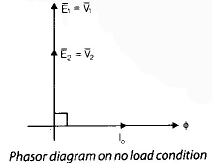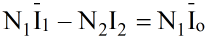⇒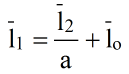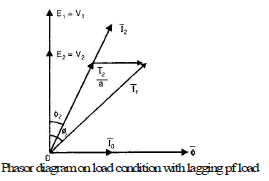Note:
• No-load current is also known as exciting current.

Case-2:
μ ≠ 0 and Core Loss Exists:
• The core losses are always present in the ferromagnetic core of the transformer since the transformer is an ac-operated magnetic device.
• The hysteresis loss in the core can be minimized by using high-grade material such as CRGO steel and eddy current loss can be minimized by using thin laminations for the core.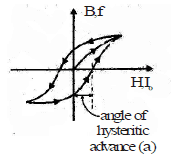• Because of hysteresis the no-load current of exciting current I0 leads the magnetic flux ø by an angle α called the angle of hysteretic advance.
Iø = I0 sin ø0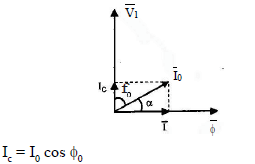• The component lø along ø is called the reactive or magnetizing current and the second component lc along V1 is called core loss component or power component.

Remember:
In an ideal transformer, core-loss current lc = 0 and no-load current l0 = magnetizing current lø.

Case-3: Effect of Leakage Flux
• The magnetic potential difference is necessary for the establishment of flux in a magnetic circuit.
• This magnetic potential difference establishes.
• The mutual flux Ø linking both the windings.
• This magnetic potential difference establishes:
- The mutual flux Ø linking both the windings.
- The primary leakage flux fl1, which links only the primary winding.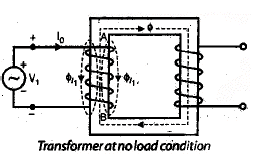• The inductor is the suitable element for showing the leakage flux element.
• Ø l1 and Øl2 are in phase with land l2 respectively because these flux are created by load current.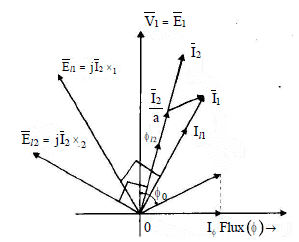• El1 and El2 are the induced emf caused by leakage fluxes in primary and secondary respectively.
• xand x2 are the leakage reactances of primary and secondary respectively.

Case-4:
Complete Phasor Diagram:
• We are using the convention that all induced EMFs and currents directly satisfy, Lenz's law (by opposing the flux).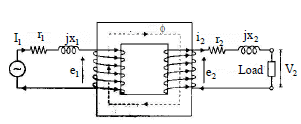Basic Equation for Transformers: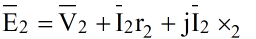•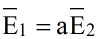•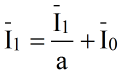•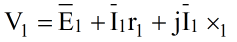•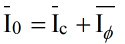Where r1 and r2 are the resistances of the windings.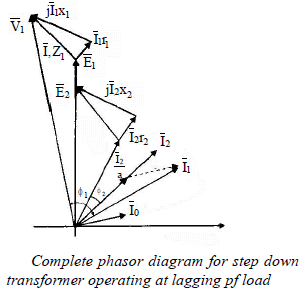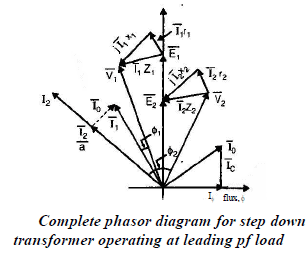Equivalent Circuit

The equivalent circuit is a circuit representation of the equation describing the performance of the device.

Exact Equivalent Circuit: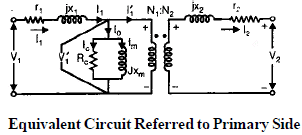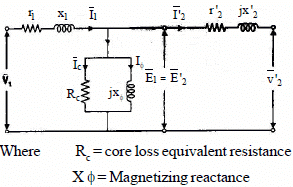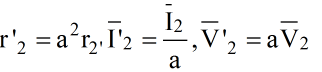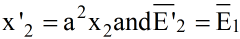• All the prime quantities are referred to the primary side.
Equivalent Circuit Referred to Secondary Side: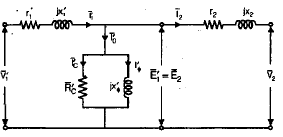• Where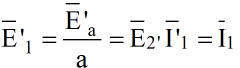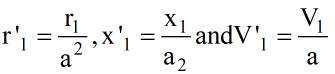Testing of Transformer

The following tests are performed to find the performance parameters of transformers.
Polarity Test
• In determining the relative polarity of the two windings of a transformer the two windings are connected in series across a voltmeter while one of the winding is excited from a suitable voltage source as shown in the figure.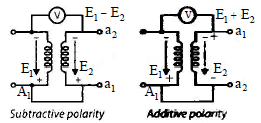• If the polarities of the windings are as marked on the diagram, the voltmeter should read E=(E1–E2). If it reads (E1 + E2), then it is called additive polarity. We don't want additive polarity because these are subjected to high voltage stresses.
• On account of these reasons, the polarity markings of one of the windings must be interchanged then after interchanging polarity voltmeter reads (E1 – E2) is called subtractive polarity. Subtractive polarity. Subtractive polarity is preferable to additive polarity.
Open-circuit (OC0 or no-load test):
• The open-circuit test given the following information:
• Core-loss at rated voltage and frequency
• The shunt branch parameters of the equivalent circuit, i.e. Rc and Xm
• Turns ratio of the transformer.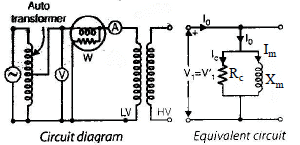• A voltmeter, wattmeter, and ammeter are connected to the low voltage side of the transformer. Readings are recorded when e applied rated frequency voltage to the L.V. side and kept high voltage side open-circuited.
• The ammeter records the no-load current or exciting current l0. Since l0 is quite small (2 to 6%) of rated current, so primary leakage impedance drop is negligible.
• The wattmeter recorded input power which consists of core loss and ohmic loss.
Remember:
The ohmic loss during the open-circuit test is negligible in composition with the normal core loss.
Parameters of the shunt branch can be found out by the following sequence of equations.

•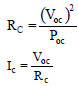•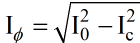•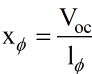Where Voc, Poc, and lo are instruments reading.
Short-circuit Test:
• The short circuit test gives the following information:
- Ohmic loss at rated current and frequency.
- The equivalent resistance and equivalent leakage reactance.
- Voltage regulation of a transformer.
Note:
• For efficiency, all data is necessary which are obtained from both test, open circuit test and short circuit test.
• In a short circuit test, all the instruments are placed on the high voltage side and the low voltage side of the transformer is short-circuited.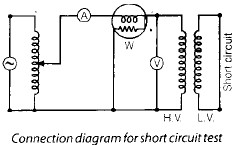• The applied voltage is adjusted by autotransformer to circulate rated current in the high voltage side.
This circulated rated current achieved by only 2 to 12% of its rated value of primary voltage.
• In this test, the wattmeter records the core loss and the ohmic loss in both the windings but the core loss and shut branch parameter ignored because only 2 to 12% of rated voltage is applied.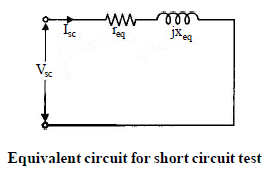• To find different parameters of transformers in the sequence of equations.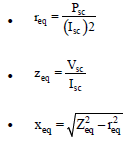Back to Back or Sumpner's test or Load Test:
• While open circuit test and short circuit tests on a transformer yield its equivalent circuit parameters, these cannot be used for the 'heat run' test wherein the purpose is to determine the steady temperature rise if the transformer was fully loaded continuously; this is so because under each of these tests the power loss to which transformer is subjected is either the core-loss or copper-loss but not both.
• In order to determine the maximum temperature rise, it is necessary to conduct a full-load test on a transformer or back-to-back test. It is also called the Regenerative test or sumpner's test. The back-to-back test on single-phase transformers requires two identical transformers.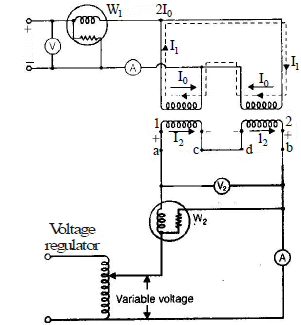• The primary windings of the two transformers are connected in parallel and supplied at rated voltage and rated frequency.
• The secondaries are connected in series with their polarities in phase opposition which can be checked by the voltmeter V2. If the voltmeter Vab across a, b point reads zero, the secondaries are in series opposition and terminal a and be are use for test.
• If voltmeter Vab =sum of the two secondaries voltages. It means these are in the same phase. In order to bring them in phase opposition, terminals ad should be joined together to result in zero voltage across terminals bc.
• The total voltage across the two secondaries in series will be zero. There will be no current in the secondary windings. The transformers will behave as if their secondary windings are open-circuited Hence, the reading of wattmeter W1 gives the iron losses of both the transformers.
• Now a small voltage is injected in the secondary circuit by a regulating transformer excited by the main supply. The magnitude of the injected voltage is adjusted till the ammeter A2 reads full load secondary current, this current produces full load current to flow through the primary windings and will follow a circulatory path through the main bus bar.
• The reading of wattmeter W2 will not be affected by this current. This wattmeter W2 given the full load copper losses of the two transformers.

Note:
• The Ammeter A1 given the total no-load current of the two transformers. Thus in this method, we have loaded the two transformers to full load but the power taken from the supply is only that necessary to supply the losses of both transformers.
• The temperature rise of transformers can be determined by operating these transformers back to back for a long time and measuring the temperature of the oil at periodic intervals of time.

The document Transformers - 2 Notes | Study Electrical Engineering SSC JE (Technical) - Electrical Engineering (EE) is a part of the Electrical Engineering (EE) Course Electrical Engineering SSC JE (Technical).
All you need of Electrical Engineering (EE) at this link: Electrical Engineering (EE)

## Electrical Engineering SSC JE (Technical)

2 videos|65 docs|37 tests
 Use Code STAYHOME200 and get INR 200 additional OFF

## Electrical Engineering SSC JE (Technical)

2 videos|65 docs|37 tests

Track your progress, build streaks, highlight & save important lessons and more!

,

,

,

,

,

,

,

,

,

,

,

,

,

,

,

,

,

,

,

,

,

;